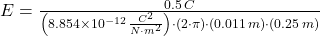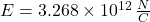## A metal, conducting cylinder of length 0.25 meters contains a total charge of 0.5 coulombs. Use Gauss’ Law to find the electric field streng

Question

A metal, conducting cylinder of length 0.25 meters contains a total charge of 0.5 coulombs. Use Gauss’ Law to find the electric field strength at a radius of 0.1 meters from the cylinder, assuming the cylinder has a radius less than 0.1 meters.

in progress 0
2 weeks 2021-08-28T18:11:54+00:00 1 Answers 0 views 0

Explanation:

Let assume that cylinder has a radius of 0.01 meters. The Gauss Law has the following form: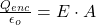The electric field considering the cylinder configuration that encloses the conducting cylinder is: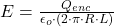Where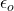is the absolute permittivity.